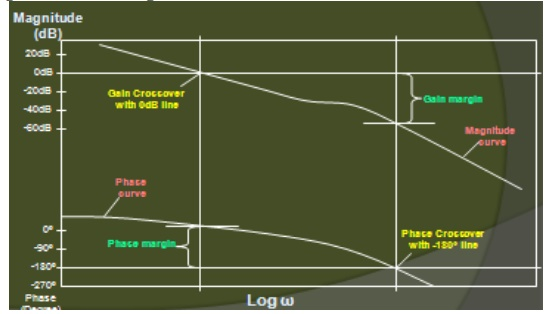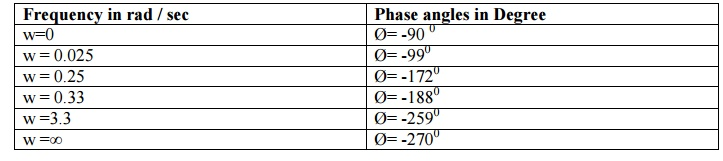# Bode plot

Plots of the magnitude and phase characteristics are used to fully describe the frequency response

Bode plot

Plots of the magnitude and phase characteristics are used to fully describe the frequency response

Bode plot is a (semilog) plot of the transfer function magnitude and phase angle as a function of frequency.

The gain magnitude is many times expressed in terms of decibels (dB)

db = 20 log 10 A

## BODE PLOT PROCEDURE:

There are 4 basic forms in an open-loop transfer function G()H()

·        Gain Factor K

·        ()±p factor: pole and zero at origin

·        (1+jωT)±q factor

1+j2ζ(W / Wn)-(W2 / Wn2)

## Gain margin and Phase margin Gain margin:

The gain margin is the number of dB that is below 0 dB at the phase crossover frequency (ø=-180º). It can also be increased before the closed loop system becomes unstable## Phase margin:

The phase margin is the number of degrees the phase of that is above -180º at the gain crossover frequency

## Gain margin and Phase margin### Bode Plot – Example

For the following T.F draw the Bode plot and obtain Gain cross over frequency (wgc) , Phase cross over frequency , Gain Margin and Phase Margin.

G(s) = 20 / [s (1+3s) (1+4s)] Solution:

The sinusoidal T.F of G(s) is obtained by replacing s by jw in the given T.F G(jw) = 20 / [jw (1+j3w) (1+j4w)]

Corner frequencies:

wc1= 1/4 = 0.25 rad /sec ;

wc2 = 1/3 = 0.33 rad /sec

Choose a lower corner frequency and a higher Corner frequency wl= 0.025 rad/sec ;

wh = 3.3 rad / sec

Calculation of Gain (A) (MAGNITUDE PLOT)

A @ wl ; A= 20 log [ 20 / 0.025 ] = 58 .06 dB

A @ wc1 ; A = [Slope from wl to wc1 x log (wc1 / wl ] + Gain (A)@wl

= - 20 log [ 0.25 / 0.025 ] + 58.06

= 38.06 dB

A @ wc2 ; A = [Slope from wc1 to wc2 x log (wc2 / wc1 ] + Gain (A)@ wc1

= - 40 log [ 0.33 / 0.25 ] + 38

= 33 dB

A @ wh ; A = [Slope from wc2 to wh x log (wh / wc2 ] + Gain (A) @ wc2 = - 60 log [ 3.3 / 0.33 ] + 33

=-27 dB

Calculation of Phase angle for different values of frequencies [PHASE PLOT] Ø = -90O- tan -1 3w – tan -1 4w

WhenFrequency in rad / sec : Phase angles in Degree

w=0 : Ø= -90 0

w = 0.025 : Ø= -990

w = 0.25 : Ø= -1720

w = 0.33 : Ø= -1880

w =3.3 : Ø= -2590

w =∞ : Ø= -2700### Calculations of Gain cross over frequency

The frequency at which the dB magnitude is Zero wgc = 1.1 rad / sec

### Calculations of Phase cross over frequency

The frequency at which the Phase of the system is - 180o wpc = 0.3 rad / sec

Gain Margin

The gain margin in dB is given by the negative of dB magnitude of G(jw) at phase cross over frequency

GM = - { 20 log [G( jwpc )] = - { 32 } = -32 dB

### Phase Margin

Ґ = 1800+ Øgc= 1800 + (- 2400o) = -600

### Conclusion

For this system GM and PM are negative in values. Therefore the system is unstable in nature.

Study Material, Lecturing Notes, Assignment, Reference, Wiki description explanation, brief detail
Control Systems : Frequency Response Analysis : Bode plot |